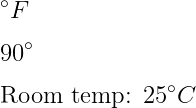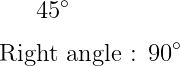# How do you write degree(a°) symbol in LaTeX?

Degree symbols are used in various fields in addition to angle measurements. Such as degree centigrade(°C), degree Fahrenheit(°F), etc.

And there is no default command to represent degree symbol in the document. However, you can create degree symbols using medium circles with superscripts. For example

\documentclass{article}
\begin{document}
$^\circ F$
$90^\circ$
Room temperature : 25 $^\circ C$
\end{document}

Output :You can convert ^\circ  syntax to a new command using the \newcommand without using direct ^\circ syntax for the degree symbol.

\documentclass{article}
\newcommand{\degree}{${#1}^\circ$}
\begin{document}
\degree{90} \\
Right angle: \degree{90}
\end{document}

Output :If you look at the code above, you will understand that you need to use the \newcommand to convert a ^\circ syntax to a degree command. And must pass \degree and ^\circ as arguments within the \newcommand command.

By default, there are no commands, but there are different commands for degree symbols in different packages.

## Use textcomp package for degree symbol

You can use the \textdegree command as an alternative to ^\circ syntax for this symbol. However, you cannot use this command in Math mode.

\documentclass{article}
\usepackage{textcomp}
\begin{document}
\textdegree C
Now temperature : 38 \textdegree C
\end{document}

Output :

      °C
Now temperature : 38 °C

## Use gensymb package for degree symbol

gensymb package contains \degree commands to represent degree symbols.

\documentclass{article}
\usepackage{gensymb}
\begin{document}
90 \degree \\
$angle{ABC} = 120 \degree$
\end{document}

Output :## Use siunitx package for degree symbols

The siunitx package contains \ang command to denote the measurement of angles and in which the magnitude of the angle must be passed as an argument. So, look at this latex program below

\documentclass{article}
\usepackage{siunitx}
\begin{document}
$\angle{ABC}=\ang{90}$
$\angle{PQR}=\ang{120}$
\end{document}

Output :And if you pass any string in the \ang command, it will show a syntax error. That is, you cannot pass any data type other than the number into the \ang command.#### Md Jidan Mondal

LaTeX expert with over 10 years of experience in document preparation and typesetting. Specializes in creating professional documents, reports, and presentations using LaTeX.